SSC CGL Previous Year Questions: Trigonometry - 2

# SSC CGL Previous Year Questions: Trigonometry - 2 - SSC CGL

Test Description

## 29 Questions MCQ Test SSC CGL (Tier - 1) - Previous Year Papers (Topic Wise) - SSC CGL Previous Year Questions: Trigonometry - 2

SSC CGL Previous Year Questions: Trigonometry - 2 for SSC CGL 2023 is part of SSC CGL (Tier - 1) - Previous Year Papers (Topic Wise) preparation. The SSC CGL Previous Year Questions: Trigonometry - 2 questions and answers have been prepared according to the SSC CGL exam syllabus.The SSC CGL Previous Year Questions: Trigonometry - 2 MCQs are made for SSC CGL 2023 Exam. Find important definitions, questions, notes, meanings, examples, exercises, MCQs and online tests for SSC CGL Previous Year Questions: Trigonometry - 2 below.
Solutions of SSC CGL Previous Year Questions: Trigonometry - 2 questions in English are available as part of our SSC CGL (Tier - 1) - Previous Year Papers (Topic Wise) for SSC CGL & SSC CGL Previous Year Questions: Trigonometry - 2 solutions in Hindi for SSC CGL (Tier - 1) - Previous Year Papers (Topic Wise) course. Download more important topics, notes, lectures and mock test series for SSC CGL Exam by signing up for free. Attempt SSC CGL Previous Year Questions: Trigonometry - 2 | 29 questions in 30 minutes | Mock test for SSC CGL preparation | Free important questions MCQ to study SSC CGL (Tier - 1) - Previous Year Papers (Topic Wise) for SSC CGL Exam | Download free PDF with solutions
 1 Crore+ students have signed up on EduRev. Have you?
SSC CGL Previous Year Questions: Trigonometry - 2 - Question 1

### If tan (A/2) = x, then the value of x is    (SSC  CHSL 2017)

Detailed Solution for SSC CGL Previous Year Questions: Trigonometry - 2 - Question 1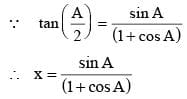SSC CGL Previous Year Questions: Trigonometry - 2 - Question 2

### If tan A = 1/2 and tan B = 1/3, then what is the value of tan (2A + B)?    (SSC  CGL 2017)

Detailed Solution for SSC CGL Previous Year Questions: Trigonometry - 2 - Question 2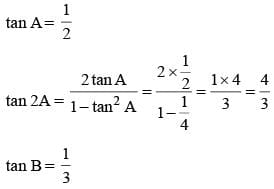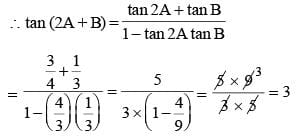SSC CGL Previous Year Questions: Trigonometry - 2 - Question 3

### What is the simplified value of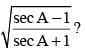(SSC  CGL 2017)

Detailed Solution for SSC CGL Previous Year Questions: Trigonometry - 2 - Question 3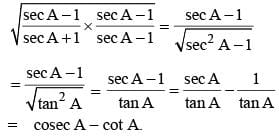SSC CGL Previous Year Questions: Trigonometry - 2 - Question 4

What is the simplified value of cosec6A – cot6A – 3 cosec2A cot2A?   (SSC  CGL 2017)

Detailed Solution for SSC CGL Previous Year Questions: Trigonometry - 2 - Question 4

cosec6A – cot6A – 3 cosec2 A cot2A
⇒ (cosec2A)3 – (cot2A)3 – 3 cot2A. cosec2A
⇒ [(cosec2A – cot2A) ((cosec2A)2 +(cot2A)2 + cosec2A cot2A)] – 3 cot2A. cosec2A
⇒ 1 [(cosec2A)2 + (cot2A)2 – 2 cosec2A cot2A + 2 cosec2A cot2A+ cosec2A cot2A)] – 3 cot2A cosec2A
⇒ [(cosec2A – cot2A)2 + 3 cosec2A cot2A] – 3 cosec2A cot2A
⇒ (cosec2A – cot2A)2 = (1)2 = 1.

SSC CGL Previous Year Questions: Trigonometry - 2 - Question 5

What is the least value of tan2θ + cot2θ + sin2θ + cos2θ + sec2θ + cosec2θ?    (SSC  CGL 2017)

Detailed Solution for SSC CGL Previous Year Questions: Trigonometry - 2 - Question 5

tan2θ + cot2θ + sin2θ + cos2θ + sec2θ + cosec2θ
⇒ sin2θ + cos2θ + tan2θ + 1 + tan2θ + cot2θ + 1 + cot2θ
⇒ 1 + 1 + 1 + 2 tan2q + 2cot2q
⇒ 3 + 2 (tan2q + cot2q)
⇒ 3 + 2 × 2 ⇒ 3 + 4 = 7

SSC CGL Previous Year Questions: Trigonometry - 2 - Question 6

If A = 30°, B = 60° an d C = 135°, then what is the value of sin3A + cos3B +  tan3C – 3sin A cos B tan C?    (SSC  CGL 2017)

Detailed Solution for SSC CGL Previous Year Questions: Trigonometry - 2 - Question 6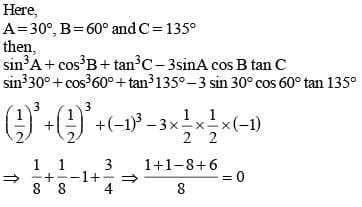SSC CGL Previous Year Questions: Trigonometry - 2 - Question 7

What is the simplified value of cosec 2A +  cot 2A?    (SSC  CGL 2017)

Detailed Solution for SSC CGL Previous Year Questions: Trigonometry - 2 - Question 7

cosec 2A + cot 2A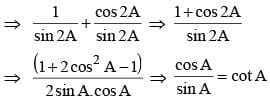SSC CGL Previous Year Questions: Trigonometry - 2 - Question 8

What is the simplified value of 1 + tan A tan (A/2)?    (SSC  CGL 2017)

Detailed Solution for SSC CGL Previous Year Questions: Trigonometry - 2 - Question 8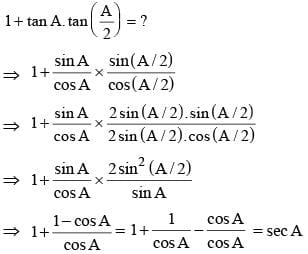SSC CGL Previous Year Questions: Trigonometry - 2 - Question 9

What is the simplified value of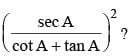(SSC  CGL 2017)

Detailed Solution for SSC CGL Previous Year Questions: Trigonometry - 2 - Question 9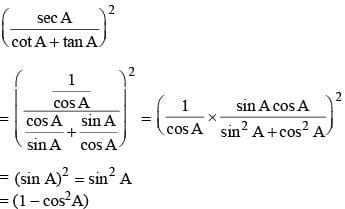SSC CGL Previous Year Questions: Trigonometry - 2 - Question 10

What is the simplified value of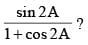(SSC  CGL 2017)

Detailed Solution for SSC CGL Previous Year Questions: Trigonometry - 2 - Question 10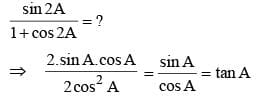SSC CGL Previous Year Questions: Trigonometry - 2 - Question 11

If cos2 θ – sinθ = 1/4, then what is the value of sin θ?    (SSC  CGL 2017)

Detailed Solution for SSC CGL Previous Year Questions: Trigonometry - 2 - Question 11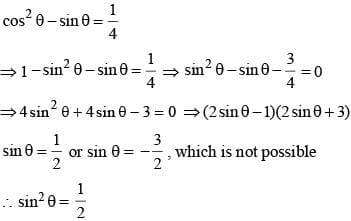SSC CGL Previous Year Questions: Trigonometry - 2 - Question 12

What is the simplified value  of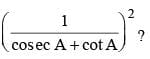(SSC  CGL 2017)

Detailed Solution for SSC CGL Previous Year Questions: Trigonometry - 2 - Question 12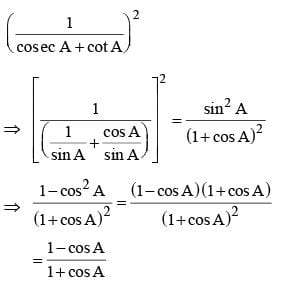SSC CGL Previous Year Questions: Trigonometry - 2 - Question 13

What is the simplified value of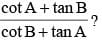(SSC  CGL 2017)

Detailed Solution for SSC CGL Previous Year Questions: Trigonometry - 2 - Question 13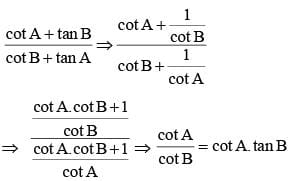SSC CGL Previous Year Questions: Trigonometry - 2 - Question 14

If tan θ + sec θ = 2, then the value of tan θ is    (SSC  Sub. Ins. 2016)

Detailed Solution for SSC CGL Previous Year Questions: Trigonometry - 2 - Question 14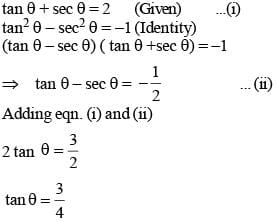SSC CGL Previous Year Questions: Trigonometry - 2 - Question 15

From the top and bottom of a straight hill, the angle of depression and elevation of the top of a pillar of 10 m. height are observed to be 60o and 30o respectively. The height (metres) of the hill is    (SSC  Sub. Ins. 2016)

Detailed Solution for SSC CGL Previous Year Questions: Trigonometry - 2 - Question 15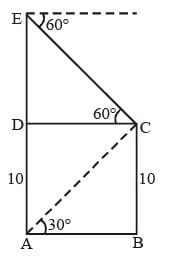Let ED = x cm
In ΔABC
AB = BC cot 30°
AB= 10√3 and
AB = DC= 10√3 and
In ΔDEC DE = DCtan60°
DE= 10√3 x √3
= 30 cm
and AE = AD + DE
=> AE = 30 + 10 = 40 cm

SSC CGL Previous Year Questions: Trigonometry - 2 - Question 16

The value of sin22o + sin24o + sin26o +..........+ sin290o is (SSC  Sub. Ins. 2016)

Detailed Solution for SSC CGL Previous Year Questions: Trigonometry - 2 - Question 16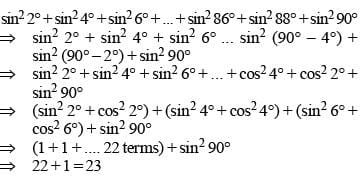SSC CGL Previous Year Questions: Trigonometry - 2 - Question 17

If 0 < A < 90o, then the value of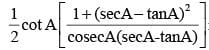is    (SSC  Sub. Ins. 2016)

Detailed Solution for SSC CGL Previous Year Questions: Trigonometry - 2 - Question 17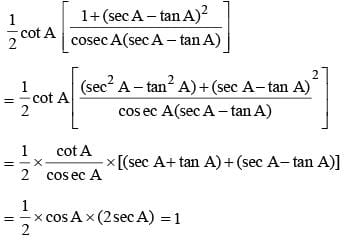SSC CGL Previous Year Questions: Trigonometry - 2 - Question 18

If 0 < θ < 90º , tanθ + sinθ = m and tan θ - sin θ = n, where m ≠ n , then value of m2 – n2 is    (SSC  Sub. Ins. 2016)

Detailed Solution for SSC CGL Previous Year Questions: Trigonometry - 2 - Question 18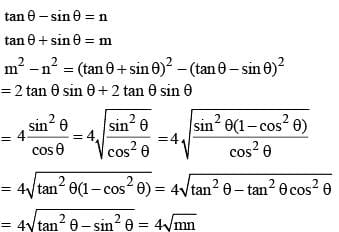SSC CGL Previous Year Questions: Trigonometry - 2 - Question 19

If cosec θ + sin θ = 5/2 then the value of cosec θ – sin θ is    (SSC CGL 1st Sit. 2016)

Detailed Solution for SSC CGL Previous Year Questions: Trigonometry - 2 - Question 19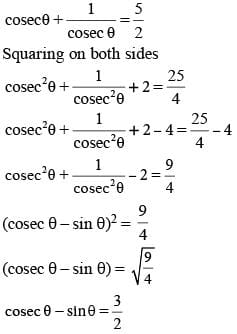SSC CGL Previous Year Questions: Trigonometry - 2 - Question 20

Value of (cos 53° – sin 37°) is    (SSC CGL 1st Sit. 2016)

Detailed Solution for SSC CGL Previous Year Questions: Trigonometry - 2 - Question 20

cos 53° – sin 37° ⇒ cos 53° – sin (90° – 53°) ⇒ cos53° – cos53° = 0

SSC CGL Previous Year Questions: Trigonometry - 2 - Question 21

Two men are on opposite sides of a tower. They measure the angles of elevation of the top of the tower as 30° and 45° respectively. If the height of the tower is 50 m, the distance between the two men is (Take √3 = 1.7)    (SSC CGL 1st Sit. 2016)

Detailed Solution for SSC CGL Previous Year Questions: Trigonometry - 2 - Question 21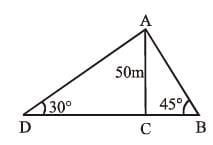AC = 50 m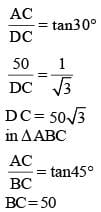BD = 50 + 50√3 = 50 (1 + √3) = 50 (2.73) = 136.5 m

SSC CGL Previous Year Questions: Trigonometry - 2 - Question 22

A pilot in an aeroplane at an altitude of 200 m observes two points lying on either side of a river. If the angles of depression of the two points be 45° and 60°, then the width of the river is    (SSC CGL 1st Sit. 2016)

Detailed Solution for SSC CGL Previous Year Questions: Trigonometry - 2 - Question 22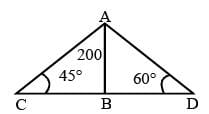Let BC be the width of river in Δ ABC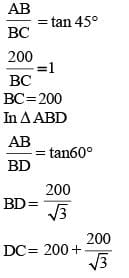SSC CGL Previous Year Questions: Trigonometry - 2 - Question 23

If tan (5x - 10°) = cot (5y + 20°), then the value of x + y is    (SSC CGL 1st Sit. 2016)

Detailed Solution for SSC CGL Previous Year Questions: Trigonometry - 2 - Question 23

tan (5x – 10°) = cot(5y + 20°)
tan (5x – 10°) = tan (90° – 5y – 20°)
5x – 10° = –5y + 70°
5x + 5y = 80°
x + y = 16°

SSC CGL Previous Year Questions: Trigonometry - 2 - Question 24

The value of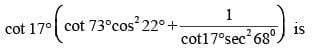(SSC CGL 1st Sit. 2016)

Detailed Solution for SSC CGL Previous Year Questions: Trigonometry - 2 - Question 24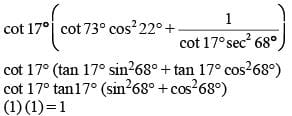SSC CGL Previous Year Questions: Trigonometry - 2 - Question 25

The upper part of a tree broke at a certain height makes an angle of 60° with the ground at a distance of 10 m. from its feet. The original height of the tree was    (SSC CGL 1st Sit. 2016)

Detailed Solution for SSC CGL Previous Year Questions: Trigonometry - 2 - Question 25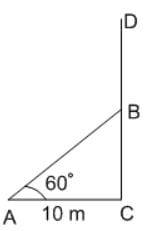Let CD be the height of the tree and it breaks from point B which touches the ground at A. As, AB = BD. Thus, Total height of the tree CD = (BC + AB) cm
In ΔABC,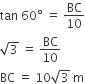Again in ΔABC,
(AB)2 = (BC)2 + (AC)2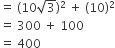(AB)2 = 400
AB = 20 cm.
∴  Original height of the tree  = AB + BC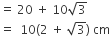SSC CGL Previous Year Questions: Trigonometry - 2 - Question 26

If θ > 0, be an acute angle, then the value of θ in degrees satisfying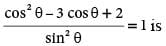(SSC CGL 1st Sit. 2016)

Detailed Solution for SSC CGL Previous Year Questions: Trigonometry - 2 - Question 26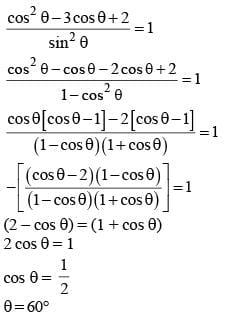SSC CGL Previous Year Questions: Trigonometry - 2 - Question 27

A tower is 50 meters high. Its shadow is x metres shorter when the sun’s altitude is 45° than when it is 30°. The value of x in metres is    (SSC CGL 1st Sit. 2016)

Detailed Solution for SSC CGL Previous Year Questions: Trigonometry - 2 - Question 27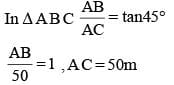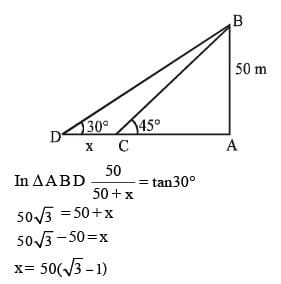SSC CGL Previous Year Questions: Trigonometry - 2 - Question 28

If sin 31° = x/y. The value of sec 31° – sin 59° is    (SSC CGL 1st Sit. 2016)

Detailed Solution for SSC CGL Previous Year Questions: Trigonometry - 2 - Question 28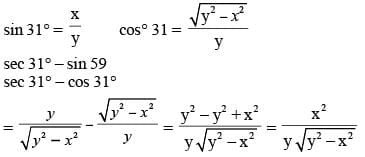SSC CGL Previous Year Questions: Trigonometry - 2 - Question 29

The circular measure of the included angle formed by the hour hand and minute hand of a clock at 3 PM will be    (SSC CGL 1st Sit. 2016)

Detailed Solution for SSC CGL Previous Year Questions: Trigonometry - 2 - Question 29

Hour hand covered in 12 hr = 360°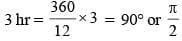## SSC CGL (Tier - 1) - Previous Year Papers (Topic Wise)

250 tests
Information about SSC CGL Previous Year Questions: Trigonometry - 2 Page
In this test you can find the Exam questions for SSC CGL Previous Year Questions: Trigonometry - 2 solved & explained in the simplest way possible. Besides giving Questions and answers for SSC CGL Previous Year Questions: Trigonometry - 2, EduRev gives you an ample number of Online tests for practice

250 tests